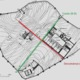•I haven't studied this at all yet, just figured I'd check the ARE handbook for an equation and take a shot at it.

Using the equation that will be provided during the test which you can find in the ARE Handbook, pg 173
https://www.ncarb.org/sites/default/files/ARE5-Handbook.pdf

a = SAC x S

a = 65 sabins

SAC = this will be one of the values from the absorption characteristics chart I'm assuming.  Idk which, I'm going to guess since the question doesn't specify, I'm going to go for the minimum sqft of panels needed, so that means going for the largest SAC value, 1.12.  though maybe I need to go with the lowest SAC of all the frequencies for a specific finish choice.  It that is the case, it looks like Heavy Spray Paint has the highest low, 0.58.  Idk.  Maybe I only need to focus on 250-2000 Hz since in the ARE handbook the equation for NRC is the avg SAC of those frequencies.

so, plug that into the equation
65 = 1.12 x S
S = 58.03 sqft

each 36" Hexus panel is 5.7 sqft.
58.03/5.7 = 10.2, round up to 11 panels.

That's my guess, I haven't studied this at all yet, just figured I'd check the ARE handbook for an equation and take a shot at it.

•Just an updated after doing some further reading.

The frequencies considered for the NRC can be used in less critical situations.  All frequencies should be used for more critical situations

Also, it appears the sum of individual materials is the total for the room, so each 5.7 sqft panel is treated the same.

•Hi,

As Luke mentioned I think we are missing some information about the problem. But, given what I know so far about this scenario, I would use the highest NRC value to calculate unless we are given preferred finishes or, for some reason, a specific frequency band (though I would not really expect that for in-room acoustical panels).

A

•From reading the product description, the feature of this product is that one can 'lightly spray paint' to match any room color without compromising its performance.

Therefore, I'll use the NRC 1.05 for this problem. (NRC is based on 250-2000 Hz range suitable for common situation covering all the middle frequencies).

65 Sabins / 1.05 NRC = 62 sq.ft

62 sq.ft / 5.7 sq.ft (36" Hexus) = 11 panels

Josh

•Josh,

Are you using the same formula that Luke suggested?

a=SAC x S

•Angela,

Yes that's correct. NRC represents the average SAC covering the standard frequency bands so it can be plugged into the formula as he's shown above. The highest NRC value would yield the best absorption, and therefore the most efficient solution.

A